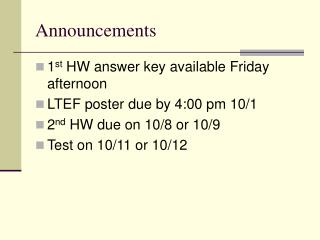DownloadDownload PresentationAnnouncements

# Announcements

Télécharger la présentation## Announcements

- - - - - - - - - - - - - - - - - - - - - - - - - - - E N D - - - - - - - - - - - - - - - - - - - - - - - - - - -
##### Presentation Transcript

1. Announcements • 1st HW answer key available Friday afternoon • LTEF poster due by 4:00 pm 10/1 • 2nd HW due on 10/8 or 10/9 • Test on 10/11 or 10/12

2. Change of State Vapor Pressure

3. What is Vaporization? • aka Evaporation • Change of state: liquid to gas

4. Is Evaporation Exo- or Endothermic? • Endothermic…why? • Must overcome the intermolecular forces • DH(vap) for H2O is 40.7 kJ/mol

5. Practical Uses for Vaporization • Most of the energy from the sun is used to evaporate water rather than warm the planet. • Evaporation cools the human body.

6. What is the opposite of vaporization? • Condensation • Rate of evaporation vs. condensation

7. Vapor Pressure • Pressure of the vapor above a liquid when the rate of evaporation and condensation are equal.

8. Measuring Vapor Pressure

9. Measuring Vapor Pressure • Different liquids have different vapor pressures. Why? • How does temperature affect vapor pressure?

10. For H2O: T (ºC)P(torr) 0.0 4.58 25.0 23.76 40.0 55.32 70.0 233.7 90.0 525.8 As T increases, vapor pressure increases What happens when T gets 100ºC? Boiling point: T at which the vapor pressure equals atm pressure. Temperature vs. Vapor Pressure

11. Graphing Pvap vs. Temperature

12. Chemistry and Straight Lines

13. Chemistry and Straight Lines • Slope: - DHVAP / R • lnP = [-DH / R][1/T] + b • lnPT1 – lnPT2 = [DH / R][1/T2 – 1/T1]

14. Clausius Clapeyron Equation ln[PT1 / PT2] = [DH / R][1/T2 – 1/T1]

15. Practice Problem • The vapor pressure of water at 25ºC is 23.8 torr and the heat of vaporization is 40.7 kJ/mol. Calculate the vapor pressure of water at 50ºC.

16. Heating Curve

17. Practice Problem 2 Calculate the enthalpy change associated with converting 1.00 mol of ice at –25.0 ºC to water vapor at 125 ºC and 1 atm pressure. c(ice) = 2.09 J / g °C DH(fus) = 6.01 kJ/mol c(liquid) = 4.18 DH(vap) = 40.7 kJ/mol c(steam) = 1.84

18. Phase Diagrams

19. What’s on the test? • Multiple choice (~20 points) • Ordering (~15 points) • Free response (~65 points) • Heating curve calculations • Phase diagram interpretations • Clausius Clapeyron manipulations • Unit cell calculations • Short answer questions